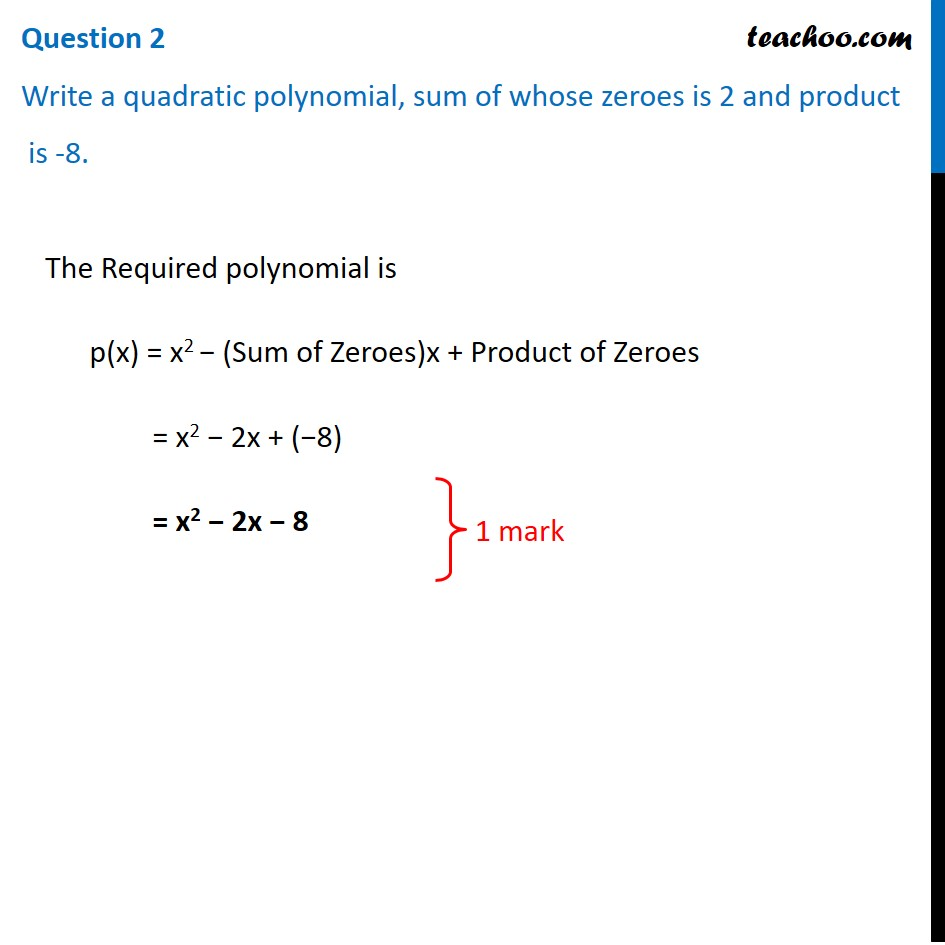## Write a quadratic polynomial, sum of whose zeroes is 2 and product is -8.1. Class 10
2. Solutions of Sample Papers for Class 10 Boards
3. CBSE Class 10 Sample Paper for 2021 Boards - Maths Basic

Transcript

Question 2 Write a quadratic polynomial, sum of whose zeroes is 2 and product is -8. The Required polynomial is p(x) = x2 − (Sum of Zeroes)x + Product of Zeroes = x2 − 2x + (−8) = x2 − 2x − 8 1 mark

CBSE Class 10 Sample Paper for 2021 Boards - Maths Basic

Class 10
Solutions of Sample Papers for Class 10 Boards